Next: Satellite orbits Up: Orbital motion Previous: Gravity

## Gravitational potential energy

We saw earlier, in Sect. 5.5, that gravity is a conservative force, and, therefore, has an associated potential energy. Let us obtain a general formula for this energy. Consider a point object of mass, which is a radial distancefrom another point object of mass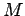. The gravitational force acting on the first mass is of magnitude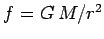, and is directed towards the second mass. Imagine that the first mass moves radially away from the second mass, until it reaches infinity. What is the change in the potential energy of the first mass associated with this shift? According to Eq. (155),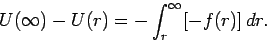(550)

There is a minus sign in front of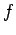because this force is oppositely directed to the motion. The above expression can be integrated to give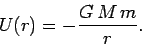(551)

Here, we have adopted the convenient normalization that the potential energy at infinity is zero. According to the above formula, the gravitational potential energy of a masslocated a distancefrom a massis simply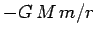.

Consider an object of massmoving close to the Earth's surface. The potential energy of such an object can be written(552)

where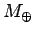andare the mass and radius of the Earth, respectively, andis the vertical height of the object above the Earth's surface. In the limit that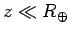, the above expression can be expanded using the binomial theorem to give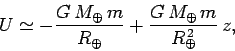(553)

Since potential energy is undetermined to an arbitrary additive constant, we could just as well write(554)

whereis the acceleration due to gravity at the Earth's surface [see Eq. (548)]. Of course, the above formula is equivalent to the formula (125) derived earlier on in this course.

For an object of massand speed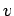, moving in the gravitational field of a fixed object of mass, we expect the total energy,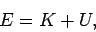(555)

to be a constant of the motion. Here, the kinetic energy is written, whereas the potential energy takes the form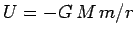. Of course,is the distance between the two objects. Suppose that the fixed object is a sphere of radius. Suppose, further, that the second object is launched from the surface of this sphere with some velocitywhich is such that it only just escapes the sphere's gravitational influence. After the object has escaped, it is a long way away from the sphere, and hence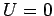. Moreover, if the object only just escaped, then we also expect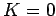, since the object will have expended all of its initial kinetic energy escaping from the sphere's gravitational well. We conclude that our object possesses zero net energy: i.e.,. Sinceis a constant of the motion, it follows that at the launch point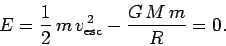(556)

This expression can be rearranged to give(557)

The quantityis known as the escape velocity. Objects launched from the surface of the sphere with velocities exceeding this value will eventually escape from the sphere's gravitational influence. Otherwise, the objects will remain in orbit around the sphere, and may eventually strike its surface. Note that the escape velocity is independent of the object's mass and launch direction (assuming that it is not straight into the sphere).

The escape velocity for the Earth is(558)

Clearly, NASA must launch deep space probes from the surface of the Earth with velocities which exceed this value if they are to have any hope of eventually reaching their targets.Next: Satellite orbits Up: Orbital motion Previous: Gravity
Richard Fitzpatrick 2006-02-02